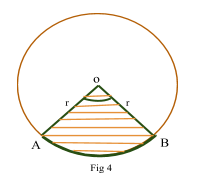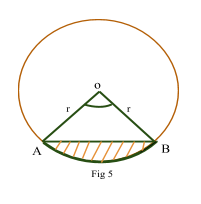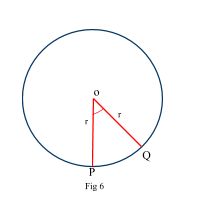Contents

### Summary

• 1 Revolution = 360°
•$\pi$ radian = 180°
• 1 radian =$\frac { 180}{ \pi }$ = 57.3°
• 1° =$\frac { \pi }{ 180 }$ radian  = 0.175 radian
• Length of arc$=\quad r\theta$
• Area of a Sector$=\quad \frac { 1 }{ 2 } { r }^{ 2 }\theta$
• Area of a segment$=\quad \frac { 1 }{ 2 } { r }^{ 2 }\theta \quad -\quad \frac { 1 }{ 2 } { r }^{ 2 }sin\theta$

The most common system of measuring the angles is that of degrees. One complete revolution is divided into 360 equal parts and each part is called one degree (1°). Furthermore, Half revolution is equivalent to$\frac { 360 }{ 2 } \quad =\quad 180$°.

Also for smaller angles, 1° is divided into 60 parts and each part is called 1 minute which is further divided into 60 parts and each part is now called 1 second.

1 Revolution = 360°
1 Degree = 60 minutes
1 minute = 60 seconds

Radians are a very important way of measuring angles. A radian is defined as the angle subtended at the centre of a circle by an arc whose length is equal to that of the radius of the circle.

The diagram ‘Fig 1’ shows a circle with centre 0 and radius r. The length of the arc AB is also r. In this position, the angle made by the arc AB at the centre is defined as 1 radian.If AB = r, then$A\hat { o } B\quad =\quad 1$  radian where$A\hat { o } B$  is the angle subtended at the center.

If AB = 2r then$A\hat { o } B\quad =\quad 2$  radian

If$AB\quad =\quad 2\pi r$  which is the circumference then$A\hat { o } B\quad$  is$2\pi$  radian. Shown in Fig 2.As$2\pi r$  is the circumference , then  OA makes a complete revolution$2\pi \quad =\quad 360$°$\pi$ radian = 180°
1 radian =$\frac {180 }{ \pi }$ = 57.3°
1° =$\frac { \pi }{ 180 }$ radian = 0.175 radian

#### Example #1

To convert an angle from degrees to radians we multiply the angle by$\frac { \pi }{ 180 }$  radian e.g:

Q. Convert 150° angle into radian.

Solution:

150°=$150\quad \times \quad \frac { \pi }{ 180 }$  radian$=\quad \frac { 5\pi }{ 6 } \quad \quad \quad rad$

#### Length of Arc

Let s be length of an arc AB which subtends an angle ???? radian at the centre O of a circle of radius r units. Shown in Fig 3.From the definition of radian, we calculate that:$\frac { arc\quad length\quad of\quad AB }{ circumference\quad of\quad the\quad circle } \quad=$$\quad \frac { Angle\quad subtended\quad by\quad AB\quad at\quad O }{ Angle\quad subtended\quad by\quad the\quad circle\quad at\quad 0 }$$\frac { s }{ 2\pi r } \quad =\quad \frac { \theta }{ 2\pi }$$s\quad =\quad r\theta$    where$\theta$  is in radians

#### Area of a sector

To find the area of the sector which contains angle ???? radian at the centre of the circleas shown in Fig 4, we consider the sector as a fraction of the circle hence:$\frac { area\quad of\quad the\quad sector\quad AOB }{ area\quad of\quad the\quad circle } \quad =$$\quad \frac { Angle\quad subtended\quad by\quad AB\quad at\quad O }{ Angle\quad subtended\quad by\quad circle\quad at\quad O }$$\frac { A }{ \pi { r }^{ 2 } } \quad =\quad \frac { \theta }{ 2\pi }$$A\quad =\quad \frac { \pi { r }^{ 2 }\theta }{ 2\pi }$$A\quad =\quad \frac { 1 }{ 2 } { r }^{ 2 }\theta$

#### Area of a segment

The area of the minor segment as shown in Fig 5is obtained by the arc AB of the centre O is given by:$Area\quad of\quad the\quad Segment\quad =\quad Area\quad of\quad the\quad sector\quad AOB\quad -\quad Area\quad of\quad the\quad triangle\quad AO$$=\quad \frac { 1 }{ 2 } { r }^{ 2 }\theta \quad -\quad \frac { 1 }{ 2 } { r }^{ 2 }sin\theta$      where$\theta$  is in radians

#### Example #2

Q. The diagram (Fig 6) shows the sector OPQ of a circle with centre O and radius r cm.The angle POQ is$\theta$  radians and the perimeter of the sector is 20 cm.

i) Show that$\theta \quad =\quad \frac { 20 }{ r } \quad -\quad 2$

ii) Find the area of the sector in terms of r.

Solution:

i) Perimeter = 20

OP + OQ + PQ =  20$r\quad +\quad r\quad +\quad r\theta \quad =\quad 20$$2r\quad +\quad r\theta \quad =\quad 20$$\theta \quad =\quad \frac { 20 }{ r } \quad -\quad \frac { 2r }{ r }$

Hence shown:$\theta \quad =\quad \frac { 20 }{ r } \quad -\quad 2$

ii) Area of the sector$OPQ\quad =\quad \frac { 1 }{ 2 } { r }^{ 2 }\theta$

Substituting$\theta$  from part (i) above$Area\quad =\quad \frac { 1 }{ 2 } { r }^{ 2 }\frac { 20 }{ r } \quad -\quad 2$

##### Reference
1. Edexcel AS and A level Modular Mathematics C2